# Aerodynamic Measurements

The following article is from The Great Soviet Encyclopedia (1979). It might be outdated or ideologically biased.

## Aerodynamic Measurements

measurements of ve locity, pressure, density, and temperature of moving air; also measurements of the forces which act on the surface of a solid body relative to which the motion takes place and measurements of the heat transfer to this surface. The majority of practical problems which are being posed for aerodynamics by aviation, rocket technology, turbine construction, industrial production, and so on require for their solution the performance of experimental studies. In these investigations the flow being examined (for example, the motion of an airplane with given altitude and velocity values) is simulated on experimental installations (wind tunnels and testing units), and the force and thermal stresses on the model being studied are determined. The maintenance of the conditions which are dictated by the theory of modeling permits the conversion of results of experiments on the model to the full-scale object. The measurement results are usually obtained as dimensionless aerodynamic coefficients that are functions of the basic similarity criteria—the Mach number, the Reynolds number, the Prandtl number, and so on—and in such a form are used for the determination of the lift and drag of the airplane, the heating of rocket and spacecraft surfaces, and so on.

Forces and moments. Measurements are made of the forces and moments which act on a body around which there is a flow. In the solution of many problems, it becomes necessary to measure the total forces which act on the model. In wind tunnels, a wind tunnel balance is generally used for the determination of the magnitudes, directions, and points of application of aerodynamic force and moment. The aerodynamic force which acts on a freely flying model can be determined by measuring the acceleration of the model. The accelerations of flying models or full-scale objects in flight tests are measured by accelerometers. If the size of the model does not permit the installation of the necessary devices, then the acceleration is found by measurement of the velocity ν of the model along a trajectory.

The total aerodynamic force (moment) which acts on a body can be represented as the sum of independent normal and tangential forces on its surface. In order to obtain the value of the normal forces, the pressure on the surface of the model is measured by means of special apertures (called drainage apertures) which are connected to manometers by rubber or metal tubing (Figure 1). The type of manometer is chosen according to the magnitude of the pressure being measured and the required accuracy of the measurements.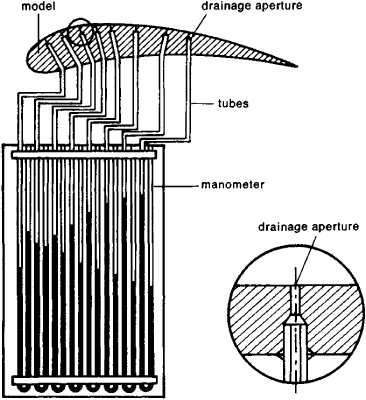Figure 1. Diagram of the measurement of static pressures on the surface of a model

If the velocity of flow around the model is so high that the compressibility of the gas is affected, it is possible to find the density distribution of the gas near the surface of the model by optical methods, and then to calculate the pressure field and obtain the pressure distribution along the model’s surface. The forces tangent to the surface of the model are usually determined by calculation; in certain cases special scales are utilized for their measurement.

Velocity of a gas flowing around a model. The velocity of gas in wind tunnels and during flow around airplanes, rockets, and flying models is measured in the majority of cases by Prandtl tubes (probes). Manometers which are connected to Prandtl tubes measure the total (p0) and static (p) pressures of the flowing gas. The velocity of an incompressible gas is determined from Bernoulli’s equation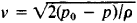where ρ is the fluid density.

If the velocity being measured is greater than the velocity of sound, a shock wave develops in front of the probe, and the reading of the manometer connected with the Pitot tube will correspond to the magnitude of the total pressure behind the shock wave: p0‘ < P0 In this case the Mach number—rather than ν—is determined (by a special formula). In the measurement of supersonic velocities, sectioned probes are usually used for the measurement of the static pressure p and the total pressure p0’ behind the normal shock wave.

There are also methods which permit the measurement of gas velocity by the variation of the amount of heat which is discharged from the heated wire of a thermoanemometer, by the ratio of densities or temperatures in stagnated and flowing gas, or by the velocity of displacement of marked particles.

For the measurement of the relatively small velocities in industrial aerodynamics and meteorology, anemometers are used; the average velocity of the gas flowing in the tube can be obtained by measuring its flow rate with special flow meters. The velocity of a flying body can also be computed by measuring the traversal time of the body through a given segment of trajectory, by the Doppler effect, and by other methods.

Gas density. The basic methods of studying the density field of a gas can be divided into three groups—methods based on the dependence of the index of light refraction on gas density, based on the absorption of radiant energy by the gas, and based on the afterglow of gas molecules in an electric discharge. The latter two groups of methods are applicable for the study of gas density at low pressure. From the first group, Töpler’s method (the “schlieren” method) and the interferometric method are used. In these methods, the dependency between the density ρ of the gas and the index of light refraction n is utilized for the measurement of the density: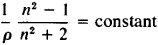In the flow of a compressible medium around a body, fields with nonuniform density distribution (density gradient fields) develop in regions where there are disturbances of the gas caused by the body around which the gas is flowing. Certain sections of the field with different density deflect light beams passing through them in different ways. A portion of the deflected beams does not pass through the focal point of the Töpler device’s detector because it is cut off by an opaque plate—the so-called Foucault knife (Figure 2); as a result, there is a local variation of the illumination of the screen (photo plate). The photographs obtained permit a qualitative analysis of the nature of the flow around the model; on these photographs regions of significant variations of density are clearly visible—shock waves, zones of rarefaction, and so on.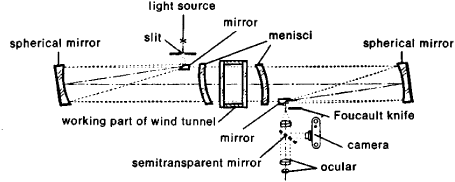Figure 2. Diagram of the Töpler device

The shock waves, which are visible on the photograph in the form of thin lines, in reality are conical surfaces on which pressure, density, and temperature discontinuities in the air take place. During flow around the ring-shaped surface of the face of the cylinder, there is a takeoff of the boundary layer from the cone’s surface.

Quantitative data concerning the density of the gas and the magnitude of the variation (gradient) of density can be obtained by comparing by a microphotometer the variation in the illumination of the screen caused by the density gradient in the flow with the variation of illumination caused by a glass reference lens which is located outside the flow of the wind tunnel; points in the flow field and on the lens which have the same brightness have the same index of refraction. From the values of the refractive index in the flow field found in the above manner, the gas density and the magnitude of the density gradient are calculated for the whole field under investigation. In addition to the photometric method, other methods for the quantitative analysis of the density field are also used.

The interferometric method of investigation of gas flow is also based on the relation between the gas density and the refractive index. The Mach-Zender interferometer is usually used for this measurement. In the photograph obtained by this method, the regions of equal illumination correspond to regions of constant density. The interpretation of the photographs permits calculation of the density in the region of flow under investigation.

One of the important advantages of optical methods is the possibility of studying gas flows without using probes and tubes of various types which are sources of disturbance in the flow.

Temperature. In a high-velocity flow, two temperatures are usually considered—that of the undisturbed flow T and that of the stopped flow T0 = T + v2 /2cp, where cρ is the specific thermal capacity of the gas at constant pressure in joules/(kg°K), v is in m/sec, and T and T0 are in 0k. It is obvious that T0T as v — 0. In a viscous gas flowing around a solid surface, the velocity on the wall is equal to zero and any immovable tube which is mounted in the airstream measures a temperature close to the stagnation temperature Ta. The readings of the device are corrected for the presence of heat leakage and such.

By means of tubes (Figure 3) in which the measuring element is usually a thermocouple or resistance thermometer, it is possible to measure a temperature T0 ≤ 1500 °K. For the measurement of higher temperatures of the stagnated or flowing gas, optical-luminosity and spectral methods are used.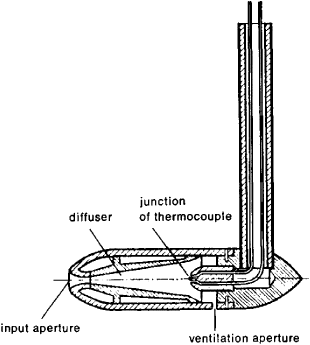Figure 3. Adaptor for measurement of the temperature of a retarded flow

The static temperature T can be found from the relation of temperature and the velocity of sound, since a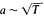For the measurement of the velocity of sound, a source of acoustic vibrations of known frequency is mounted on the wall of a wind tunnel. The sound waves will be visible in the shadow photograph of the flow field. The velocity of sound is defined as a = fe, where e is the distance between waves and f is the frequency of the vibrations of the source (Figure 4).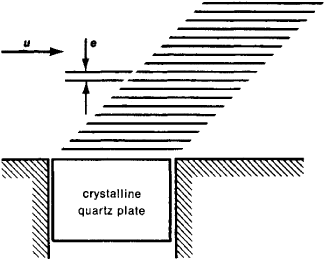Figure 4. Diagram of the measurement of gas temperature as a function of the speed of propagation of acoustical waves

Tangential forces (friction) and thermal fluxes on the surface of a model. For the determination of the shearing stresses τ and thermal flux q, it is possible to perform the measurement of velocity and temperature fields of a gas near the surface and find the unknown values by using the Newton equation for frictional stresses τ = μ (dvldy) and the equation of heat conduction q = λ (dT/dy) where μ and λ are the coefficients of dynamic viscosity and heat conductance of the gas, and dvfdy and dT/dy are the gradients of velocity and temperature near the surface of the body in the direction y, normal to the surface. Practically, it is impossible to obtain with sufficient accuracy the values dvldy and dT/dy as y → 0. Therefore, for the determination of the frictional forces and heat fluxes from the measurement of the velocity and temperature fields in the boundary layer, so-called integral methods are applied. In these methods the frictional force and the heat flow on the section of the surface under consideration are determined by measurements of the thickness of the boundary layer and the velocity and temperature profiles.

More accurate values for τ and q can be obtained by direct measurement. To do this, the tangential force ΔX on an element of surface ΔS is measured by special balances; the shearing stresses are defined as τ = ΔX/Δ S. Similarly, using calorimeters of various types, it is possible to measure the thermal flux q which flows through the element of the surface ΔS under consideration and to obtain the specific thermal flux q* = q/Δ S. To obtain the distribution of thermal fluxes along the surface of a body, one usually determines the rate of temperature rise dT/dt, which is measured by thermocouples mounted in special calorimeters built into the surface of the model, or by thermocouples which are soldered into the thin surface of the model with comparatively small heat conductance.

The increase of the altitude and velocity of flight and the necessity of modeling processes which develop behind intense shock waves and close to the body’s surface led to the widespread use in aerodynamic experimentation of other physical methods of measurement—for example, spectral methods applicable to shock tubes, radioisotope methods for the measurement of the breakdown rate of heat-shielding materials, methods of measuring the electric conductivity of a gas which is being heated by a shock wave, and so on.

### REFERENCES

Popov, S. G. Izmerenie vozdushnykh potokov. Moscow-Leningrad, 1947.
Popov, S. G. Nekotorye zadachi i melody eksperimental’noi aeromekhaniki. Moscow, 1952.
Pankhurst, R., and D. Holder. Tekhnika eksperimenta ν aerodinamicheskikh trubakh. Moscow, 1955. (Translated from English.)
Ladenburg, R., D. Winkler, and K. van Wooris. “Izuchenie sver-khzvukovykh iavlenii pri pomoshchi interferometra.” Voprosy raketnoi tekhniki, 1951, issues 1–2.
Tekhnika giperzvukovykh issledovanii. Moscow, 1964. (Translated from English.)
Aerofizicheskie issledovaniia sverkhzvukovykh techenii. Moscow-Leningrad, 1966.
Sovremennaia tekhnika aerodinamicheskikh issledovanii pri giperzvukovykh skorostiakh. Edited by A. Krill. Moscow, 1965. (Translated from English.)

M. IA. IUDELOVICH